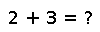# Set

``````..  Set([itr])

Construct a :obj:`Set` of the values generated by the given iterable object, or an empty set.
Should be used instead of :obj:`IntSet` for sparse integer sets, or for sets of arbitrary objects.``````

## Examples

``````julia> empty_set = Set()
Set{Any}({})``````
``````julia> filledSet =  Set(1,2,2,3)
Set{Int64}({2,3,1})``````
``````julia> filledSet = Set(1,2,3)
Set{Int64}({2,3,1})``````

Checking you are not a robot: